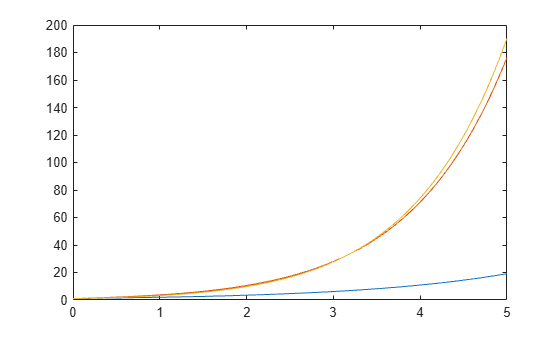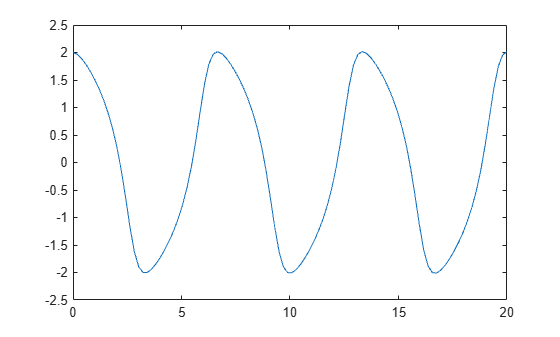Main Content

# deval

Evaluate differential equation solution structure

## Syntax

``y = deval(sol,x)``
``````y = deval(x,sol)``````
``y = deval(___,idx)``
``````[y,yp] = deval(___)``````

## Description

example

````y = deval(sol,x)` and ```y = deval(x,sol)``` evaluate the solution `sol` of a differential equation problem at the points contained in `x`.```

example

````y = deval(___,idx)` returns only the solution components with indices listed in the vector `idx`. You can use either of the previously listed input argument combinations.```

example

``````[y,yp] = deval(___)``` also returns `yp`, which is the first derivative of the numeric solution produced by the solver.```

## Examples

collapse all

This example solves the DDE equation `y' = ddex1de(t,y,Z)` using `dde23`, then plots the solution.

Solve the system using `dde23`.

`sol = dde23(@ddex1de, [1 0.2], @ddex1hist, [0 5]);`

Evaluate the solution at 100 points in the interval `[0 5]`.

```x = linspace(0,5); y = deval(sol,x);```

Plot the solution.

`plot(x,y)`This example solves the system `y' = vdp1(t,y)` using `ode45`, then plots the first component of the solution.

Solve the system using `ode45`.

`sol = ode45(@vdp1, [0 20], [2 0]);`

Evaluate the first component of the solution at 100 points in the interval `[0 20]`.

```x = linspace(0,20,100); y = deval(sol,x,1);```

Plot the solution.

`plot(x,y)`Solve the simple ODE `y' = t^2` with initial condition `y0 = 0` in the interval $\left[0,3\right]$ using `ode23`.

`sol = ode23(@(t,y) t^2, [0 3], 0);`

Evaluate the solution at seven points. The solution structure `sol` contains an interpolating function that `deval` uses to produce a continuous solution at these points. Specify a second output argument with `deval` to also return the derivative of the interpolating function at the specified points.

```x = linspace(0,3,7); [y,yp] = deval(sol,x)```
```y = 1×7 0 0.0417 0.3333 1.1250 2.6667 5.2083 9.0000 ```
```yp = 1×7 0 0.2500 1.0000 2.2500 4.0000 6.2500 9.0000 ```

## Input Arguments

collapse all

Solution structure, specified as a structure returned by one of these differential equation solvers.

• Initial value problem solver — `ode45`, `ode23`, `ode113`, `ode15s`, `ode23s`, `ode23t`, `ode23tb`, `ode15i`

• Delay differential equations solver — `dde23`, `ddesd`, or `ddensd`

• Boundary value problem solver — `bvp4c` or `bvp5c`

Example: `sol = ode45(@myode,tspan,y0)`

Data Types: `struct`

Evaluation points, specified as a vector. `x` specifies the points at which you want the value of the solution. The elements of `x` must be contained in the original integration interval, `[sol.x(1) sol.x(end)]`. For each index `i`, the solution `y(:,i)` corresponds to `x(i)`.

Example: `0:0.1:1`

Example: ```[2 3 5 8]```

Data Types: `single` | `double`

Solution components to return, specified as a vector. Use this input when you are only interested in certain components of the solution.

Example: `y = deval(sol,x,[2 3])` returns only the second and third solution components.

Data Types: `single` | `double`

## Output Arguments

collapse all

Interpolated solution, returned as a vector or matrix. The number of rows in `y` is equal to the number of solution components being returned.

For multipoint boundary value problems, the solution obtained by `bvp4c` or `bvp5c` might be discontinuous at the interfaces. For an interface point `xc`, the `deval` function returns the average of the limits from the left and right of `xc`. To get the limit values, set the value of `x` to be slightly larger or smaller than `xc`.

Derivative of continuous solution produced by `sol`, returned as a vector or matrix. `yp` is the same size as `y` and indicates the slope of the interpolating function used by `sol` at each solution point in `y`.

## See Also

Introduced before R2006a

Download ebook